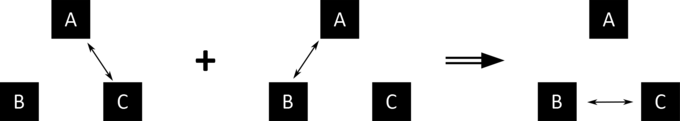# Zeroth Law of Thermodynamics - Thermal Equilibrium

### Zeroth Law of Thermodynamics:

Before the zeroth law of thermodynamics was put forward, there had already existed 3 laws of Thermodynamics. These are:

• 1st Law of Thermodynamics
• 2nd Law of Thermodynamics
• 3rd Law of Thermodynamics

Scientists came to a realization that these 3 sets of laws aren't exactly complete with regards to temperature. They envisaged the need for a law that will introduce a formal definition of temperature.

Due to the nature and importance of this law, it needed to supersede the three existing laws. At that time, the three laws (named as 1st, 2nd and 3rd respectively) were already popular in the academic world. Since these three laws are widely known by their numbers, a numbering confusion came up.

The alternative of calling this new law the Fourth Law woundn't be ideal due to it's position and importance. It was Ralph Fowler, a scientist that called the new law the “Zeroth Law" which was a naming caveat that has become popular to this very day.

The Zeroth Law of Thermodynamics states that if two separate bodies are in thermal equilibrium with another body, then they are also in equilibrium with each other.

To better understand what the zeroth law meant, we need to know what thermal equilibrium is;

Two bodies A and B are said to be in thermal equilibrium if each body can transfer heat to each other when placed closed together but ended up NOT transferring any heat to each other.

This will mean that both bodies are at equal temperature.

Now, according to the zeroth law, assuming we have three bodies namely X, Y and Z:

if X = Y and Z = Y, then X = Z

From the above expression, it will be observed that: if X and Y are in thermal equilibrium and Z and Y are in thermal equilibrium, therefore, X and Z must be in thermal equilibrium too.

So we can see that according to the zeroth law, Temperature is a quantity worth measuring.

When we put a pot of water on a cooking gas, we will notice the transfer of heat from the cooking gas to water since the water begins to get warm.

As heat transfer continues, the water becomes warmer and eventually becomes hot. A point will be reached when the water starts to boil. (The boiling boiling point of pure water is 100OC).

Thermal equilibrium for the pure water is reached at 100OC because the water cannot become hotter; instead, it evaporates as water vapour.

Although, Temperature is not directly mentioned in the zeroth law, the law actually points us towards the existence of temperature as a quantity.

It can therefore be stated that Temperature is the quantity that remains constant (the same) for all systems in thermal equilibrium.Alfred Ajibola is a Medical Biochemist, a passionate Academician with over 7 years of experience, a Versatile Writer, a Web Developer, a Cisco Certified Network Associate and a Cisco CyberOps Associate.

#### NOTABLE POINTS IN Physics Fuses are present in many electrical devices. They are basically 🔥 fire-breakers 🔥 If there is a power surge, the fuse will break, preventing damage to the electrical devices. It will also prevent the incidence of 🔥 fire🔥 on/from such device. Physics is one of the core sciences. Physics is defined the scientific study of matter and the movement of matter through the space and time of the universe. Understanding the basic and fundamental concepts of physics will help you better comprehend this subject. Below are some of the concepts: The Laws of Motion (Classical Mechanics) Electromagnetism Relativity Thermodynamics Please read on the basic introduction of the above physics concept here

CONTRIBUTE TO THIS TOPIC | ASK A QUESTION